# Compare Fixed-Point Viterbi Decoding to Theoretical Calculations

Use the Viterbi Decoder block for fixed-point hard- and soft-decision convolutional decoding. Compare results to theoretical upper bounds as computed with the Bit Error Rate Analysis app.

### Simulation Configuration

The `cm_viterbi_harddec_fixpt` and `cm_viterbi_softdec_fixpt` models highlight the fixed-point modeling attributes of the Viterbi decoder, using similiar layouts. The default configuration of the models use the `PreLoadFcn` Callback to specify an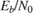setting of 4 dB for the AWGN Channel block. The convolutional encoder is configured as a rate 1/2 encoder. Specifically, for every 2 bits the encoder adds another 2 redundant bits. To accommodate the code rate, the Eb/No (dB) parameter of the AWGN block is halved by subtracting `10*log10(2)` from the assignedsetting. To limit the run duration to 100 errors or 1e6 bits for the Error Rate Calculation block.

### Fixed-Point Modeling

Fixed-point modeling enables bit-true simulations which take into account hardware implementation considerations and the dynamic range of the data and parameters. For example, if the target hardware is a DSP microprocessor, some of the possible word lengths are 8, 16, or 32 bits, whereas if the target hardware is an ASIC or FPGA, there may be more flexibility in the word length selection.

To enable fixed-point Viterbi decoding,

• For hard decisions, the block input must be of type ufix1 (unsigned integer of word length 1). Based on this input (either a 0 or a 1), the internal branch metrics are calculated using an unsigned integer of word length = (number of output bits), as specified by the trellis structure (which equals 2 for the hard-decision example).

• For soft decisions, the block input must be of type ufixN (unsigned integer of word length N), where N is the number of soft-decision bits, to enable fixed-point decoding. The block inputs must be integers in the range 0 to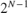. The internal branch metrics are calculated using an unsigned integer of word length = (N + number of output bits - 1), as specified by the trellis structure (which equals 4 for the soft-decision example).

The State metric word length is specified by the user and usually must be greater than the branch metric word length already calculated. You can tune this to be the most suitable value (based on hardware and data considerations) by reviewing the logged data for the system.

Enable the logging by selecting Apps > Fixed-Point Tool. In the Fixed-Point Setting menu, set the Fixed-point instruments mode to `Minimums, maximums and overflows`, and rerun the simulation. If you see overflows, it implies the data did not fit in the selected container. You could either try scaling the data prior to processing it or, if your hardware allows, increase the size of the word length. Based on the minimum and maximum values of the data, you are also able to determine whether the selected container is of the appropriate size.

Try running simulations with different values of State metric word length to get an idea of its effect on the algorithm. You should be able to narrow down the parameter to a suitable value that has no adverse effect on the BER results.

The hard decision configuration for the `cm_viterbi_harddec_fixpt` model: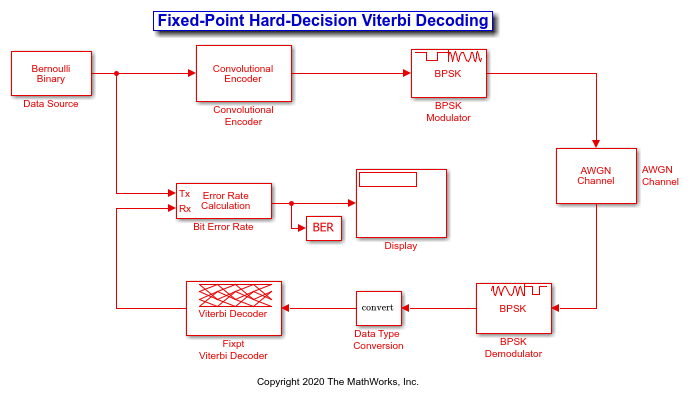• The BPSK Demodulator Baseband produces hard decisions, which are passed onto the decoder.

• The Data Type Conversion (Simulink) block sets the `Mode` parameter to `Fixed point` and casts the output data type to `fixdt(0,1,0)`. The signal input to the Viterbi Decoder block is `ufix1`.

• The Viterbi Decoder block has the `Decision type` parameter set to `Hard decision`, and on the Data Types tab, the `State metric word length` is set to `4` and the `Output data type` is set to `boolean`. The bit error rate is displayed and captured to the `BER` workspace variable.

The soft decision configuration for the `cm_viterbi_softdec_fixpt` model: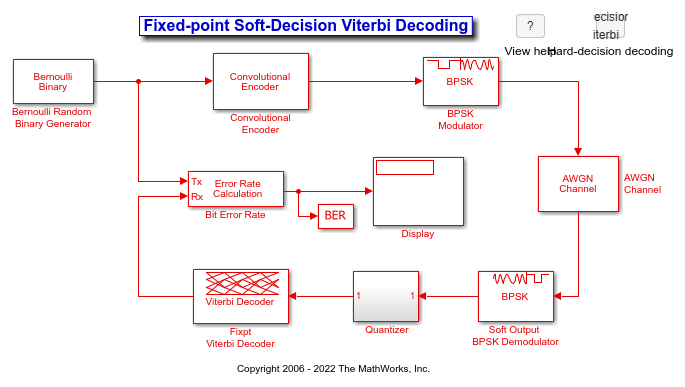• The Viterbi Decoder block has the `Decision type` parameter set to `Soft decision` and `Number of soft decision bits` set to 3, and on the Data Types tab, the `State metric word length` is set to `6` and the `Output data type` is set to `boolean`. The bit error rate is displayed and captured to the `BER` workspace variable.

### Comparisons Between Hard and Soft-Decision Decoding

The two models are configured to run from within the Bit Error Rate Analysis app to generate simulation curves to compare the BER performance for hard-decision versus soft-decision decoding.

You can produce the results in this plot by following the steps outlined below.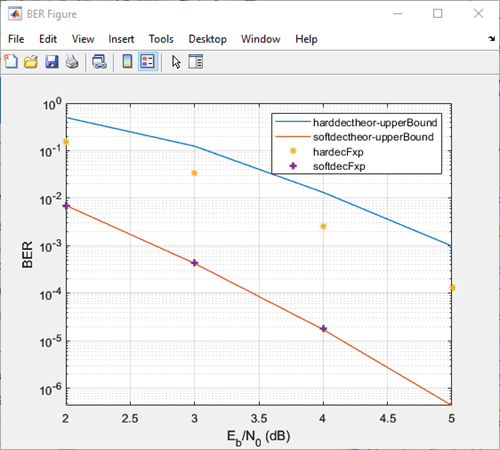These steps generate simulation results for theoretical and fixed-point hard and soft decision Viterbi decoding:

1. Open the Bit Error Rate Analysis app by selecting it in the Apps tab or by entering `bertool` at the MATLAB command prompt.

2. On the Theoretical pane, with Eb/N0 range set to 2:5, Channel type set to `AWGN`, Modulation type set to `PSK`, Channel coding set to `Convolutional`, run with Decision method set to `Hard` and then `Soft`. Rename the BER Data Set to identify the hard and soft data sets of theoretical results.

3. On the Monte Carlo pane, set Eb/N0 range to 2:1:5, for Simulation environment select `Simulink`, BER variable name set to `BER`, for Simulation limits set Number of errors to 100 and Number of bits to 1e6. Run with Model name set to `cm_viterbi_harddec_fixpt` and then `cm_viterbi_softdec_fixpt`. Rename the BER Data Set to identify the hard and soft data sets of Simulink results.

After the four runs the app will resemble this image.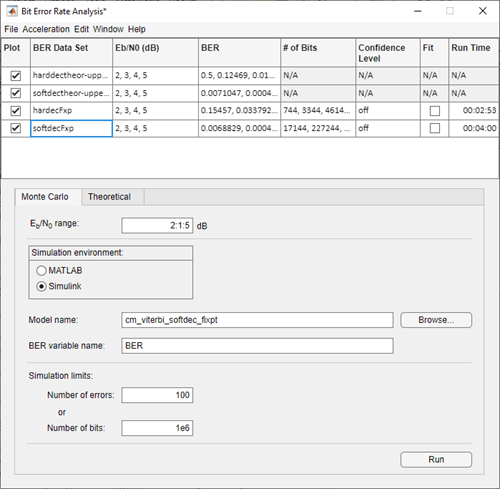### Comparisons with Double-Precision Data

For further explorations, you can run the same model with double precision data by selecting `Apps > Fixed-Point Tool`. In the `Fixed-Point Tool` app, select the `Data type override` to be `Double`. This selection overrides all data type settings in all the blocks to use double precision. For the Viterbi Decoder block, as `Output type` was set to `boolean`, this parameter should also be set to `double`.

Upon simulating the model, note that the double-precision and fixed-point BER results are the same. They are the same because the fixed-point parameters for the model have been selected to avoid any loss of precision and optimize memory efficiency.